# 1 Tuesday, January 11

References:

Emphasis for the course: applications to low-dimensional topology, lots of examples, and ways to construct contact structures. The first application is critical to 4-manifold theory:

## 1.1 Application 1

Every diffeomorphism $$f: S^3\to S^3$$ extends to a diffeomorphism $${\mathbb{B}}^4\to {\mathbb{B}}^4$$.

This isn’t true in all dimensions! This is essentially what makes Kirby calculus on 4-manifolds possible without needing to track certain attaching data.

There is a standard contact structure on $$S^3$$: regard $${\mathbb{C}}^2 \cong {\mathbb{R}}^4$$ and suppose $$f:S^3\to S^3$$. There is an intrinsic property of contact structures called tightness which doesn’t change under diffeomorphisms and is fundamental to 3-manifold topology.

There is a unique tight contact structure $$\xi_\text{std}$$ on $$S^3$$.

So up to isotopy, $$f$$ fixes $$\xi_\text{std}$$.

A useful idea: tiling by holomorphic discs. This involves taking $$S^1$$ and foliating the bounded disc by geodesics – by the magic of elliptic PDEs, this is unobstructed and can be continued throughout the disc just using convexity near the boundary. In higher dimensions: $${\mathbb{B}}^4$$ is foliated by a 2-dimensional family of holomorphic discs.

## 1.2 Application 2

Another application: monotonic simplification (?) of the unknot. Given a knot $$K \hookrightarrow S^3$$, a theorem of Alexander says $$K$$ can be braided about the $$z{\hbox{-}}$$axis, which can be described by a word $$w\in B_n$$, the braid group \begin{align*} B_n = \left\{{ \sigma_1,\cdots, \sigma_{n-1} {~\mathrel{\Big\vert}~}[\sigma_i, \sigma_j] = 1 \,{\left\lvert {i-j} \right\rvert}\geq 2,\, \sigma_i \sigma_{i+1}\sigma_i = \sigma_{i+1}\sigma_i \sigma_{i+1}\, i=1,\cdots, n-2 }\right\} .\end{align*} This captures positive vs negative braiding on nearby strands, commuting of strands that are far apart, and the Reidemeister 3 move. Write $$K = K(\beta)$$ for $$\beta$$ a braid for the braid closure.

Markov’s theorem: if $$K = K(\beta_1), K(\beta_2)$$ where $$\beta_1 \in B_n$$ and $$\beta_2\in B_m$$ with $$m,n$$ not necessarily equal, then there is a sequence of Markov moves $$\beta_1$$ to $$\beta_2$$. The moves are:

• Stabilization and destabilization: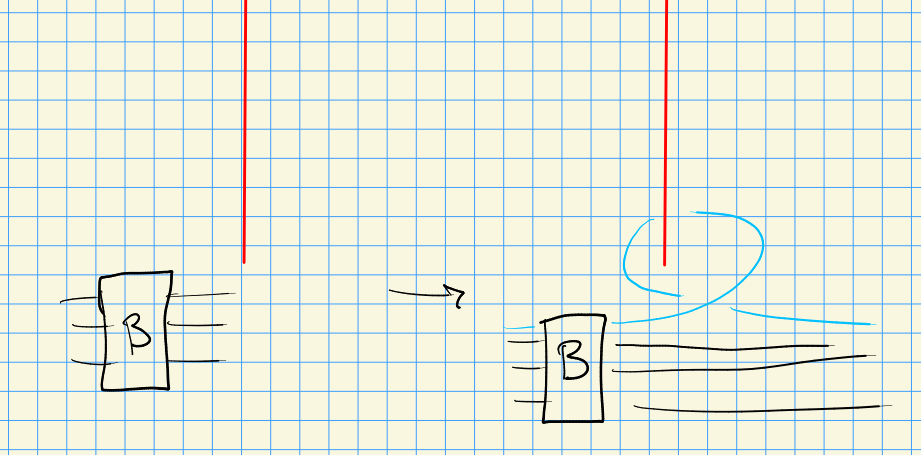• Conjugation in $$B_n$$: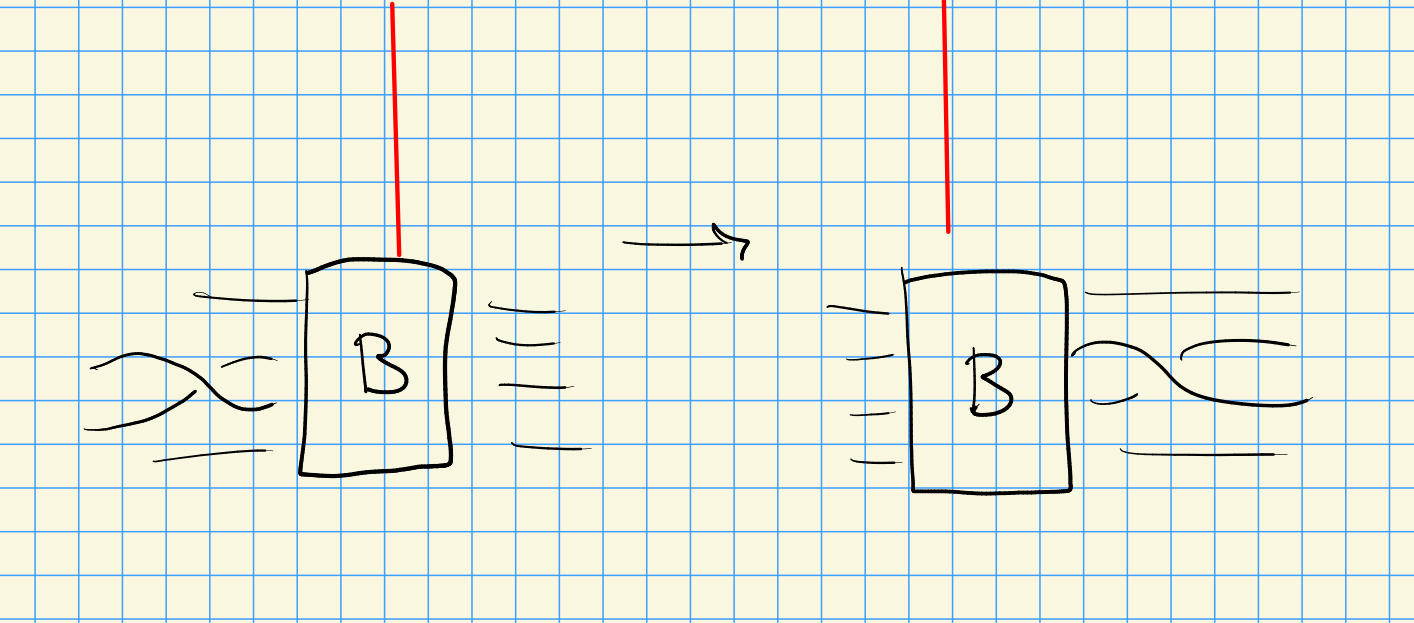• Braid isotopy, which preserves braid words in $$B_n$$.

A theorem of Birman-Menasco: if $$K(\beta) = U$$ is the unknot for $$\beta \in B_n$$, then there is a sequence of braids $$\left\{{\beta_i}\right\}_{i\leq k}$$ with $$\beta_k = 1 \in B_1$$ such that

• $$\beta_i\in B_{n_i}$$
• $$K(\beta_i) = U$$
• $$n_1\geq n_2\geq \cdots \geq n_k = 1$$
• $$\beta_i \to \beta_{i+1}$$ is either a Markov move or an exchange move.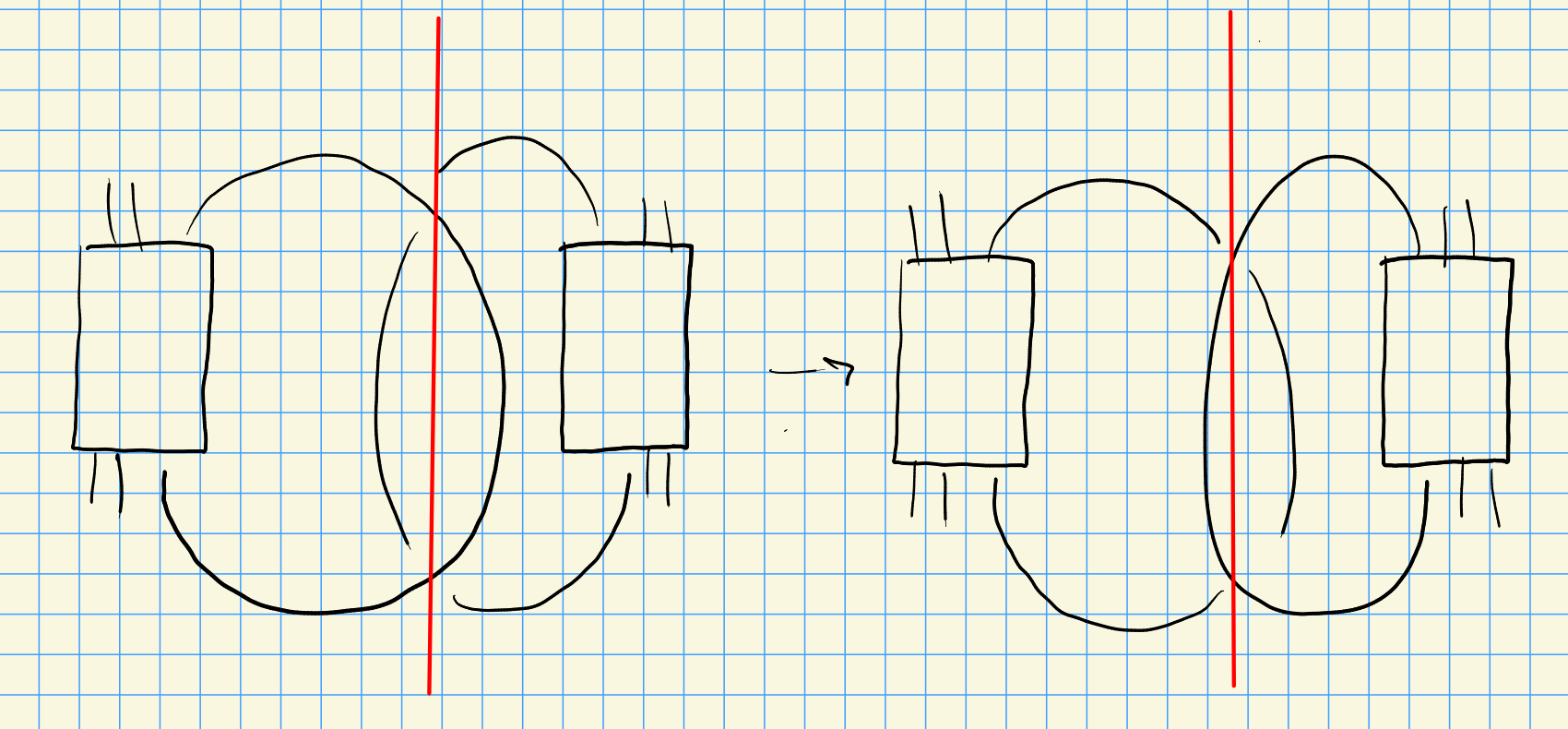## 1.3 Application 3

Genus bounds. A theorem due to Thurston-Eliashberg: if $$\xi$$ is either a taut foliation or a tight contact structure on a 3-manifold $$Y$$ and $$\Sigma \neq S^2$$ is an embedded orientable surface in $$Y$$, then there is an Euler class $$e(\xi) \in H^2(Y)$$. Then \begin{align*} {\left\lvert { {\left\langle { e(\xi)},~{ \Sigma } \right\rangle} } \right\rvert} \leq -g(\Sigma) ,\end{align*} which after juggling signs is a lower bound on the genus of any embedded surface.

Taut foliations: the basic example is $$F\times S^1$$ for $$F$$ a surface. The foliation carries a co-orientation, and the tangencies at critical points of an embedded surface will have tangent planes tangent to the foliation, so one can compare the co-orientation to the outward normal of the surface to see if they agree or disagree and obtain a sign at each critical point. Write $$c_\pm$$ for the number of positive/negative elliptics and $$h_\pm$$ for the hyperbolics. Then \begin{align*} \chi = (e_+ + e_-) - (h_+ + h_-) ,\end{align*} by Poincaré-Hopf. On the other hand, $${\left\langle {e(\xi)},~{\Sigma} \right\rangle} = (e_+ - h_+) - (e_- - h_-)$$, so adding this yields \begin{align*} {\left\langle {e(\xi)},~{\Sigma} \right\rangle} +\chi = 2(e_+ - h_+) \leq 0 .\end{align*} Isotope the surface to cancel critical points in pairs to get rid of caps/cups so that only saddles remain.

## 1.4 Contact Geometry

A contract structure on $$Y^{2n+1}$$ is a hyperplane field (a codimension 1 subbundle of the tangent bundle) $$\xi = \ker \alpha$$ such that $$\alpha \wedge (d\alpha){ {}^{ \scriptscriptstyle\wedge^{n} } } > 0$$ is a positive volume form.

On $${\mathbb{R}}^3$$, \begin{align*} \alpha = dz - ydx \implies d\alpha = -dy \wedge dx = dx\wedge dy ,\end{align*} so \begin{align*} \alpha \wedge d\alpha = (dz-ydx)\wedge(dx\wedge dy) = dz\wedge dx\wedge dy = dx\wedge dy\wedge dz .\end{align*}

On $${\mathbb{R}}^5$$, set $$\alpha = dz-y_1 dx_1 - y_2 dx_2$$. Check that \begin{align*} \alpha\wedge (d\alpha)^2 = 2(dz \wedge dx_1 \wedge dy_1 \wedge dx_2 \wedge dy_2) .\end{align*}

# 2 Contact Forms and Structures (Thursday, January 13)

A contact form on $$Y^3$$ is a 1-form $$\alpha$$ with $$\alpha \wedge d\alpha > 0$$. A contact structure is a 2-plane field $$\xi = \ker \alpha$$ for some contact form.

Forms are more rigid than structures: if $$f>0$$ and $$\alpha$$ is contact, then $$f\cdot \alpha$$ is also contact with $$\ker( \alpha ) = \ker(f \alpha)$$.

## 2.1 Examples of Contact Structures

On $${\mathbb{R}}^3$$, a local model is $$\alpha\mathrel{\vcenter{:}}=\,dz- y\,dx$$.

Show $$\alpha \wedge d\alpha = dz\wedge dx \wedge dy$$.

Write $$\xi = \mathop{\mathrm{span}}_{\mathbb{R}}({\partial}y, y {\partial}z + {\partial}x)$$, which yields planes with a corkscrew twisting. Verify this by writing $$\alpha = 0 \implies {\frac{\partial z}{\partial x}\,} = y$$, so the slope depends on the $$y{\hbox{-}}$$coordinate.

On $${\mathbb{R}}^3$$, take $$\alpha_2 \mathrel{\vcenter{:}}= dz + x\,dy$$ and check $$\alpha_2 \wedge d \alpha_2 = dz \wedge dx \wedge dy$$. This is a rigid rotation by $$\pi/2$$ of the previous $$\alpha$$, so doesn’t change the essential geometry.

Again on $${\mathbb{R}}^3$$, take $$\alpha_3 = dz + {1\over 2}r^2 d\theta$$. Check that $$d \alpha_3 = r\,dr\wedge \,d\theta$$ and $$\alpha_3 \wedge d \alpha_3 = r\,dz\wedge \,dr\wedge \,d\theta$$. Then $$\xi = \mathop{\mathrm{span}}_{\mathbb{R}}({\partial}r, {1\over 2} r^2 {\partial}z + {\partial}\theta )$$. Note that as $$r\to \infty$$, the slope of these planes goes to infinity, but doesn’t depend on $$z$$ or $$\theta$$.

Set $$\alpha_4 = \,dz+ {1\over 2}(x\,dy- y\,dx)$$, then this is equal to $$\alpha_3$$ in rectangular coordinates.

Set \begin{align*} \alpha_4 = \cos(r^2)\,dz+ \sin(r^2)\,d\theta .\end{align*}

Compute the exterior derivative and check that this yields a contact structure.

Now note that \begin{align*} \alpha = 0 \implies {\frac{\partial z}{\partial \theta}\,} = -{\sin(r^2)\over \cos(r^2)} = -\tan(r^2) ,\end{align*} which is periodic in $$r$$. So a fixed plane does infinitely many barrel rolls along a ray at a constant angle $$\theta_0$$.

This is far too twisty – to see the twisting, consider the graph of $$(r, \tan(r^2))$$ and note that it flips over completely at odd multiples of $$\pi/2$$. In the previous examples, the total twist for $$r\in (-\infty, \infty)$$ was less than $$\pi$$.

A contactomorphism is a diffeomorphism \begin{align*} \psi: (Y_1^3, \xi_1) \to (Y_2^3,\xi_2) \end{align*} such that $$\phi_*(\xi_1) = \xi_2$$ (tangent vectors push forward).

A strict contactomorphism is a diffeomorphism \begin{align*} \phi: (Y_1^3, \ker \alpha_1) \to (Y_2^3, \ker \alpha_2) .\end{align*} such that $$\phi^*( \alpha_2) = \alpha_1$$ (forms pull back).

Strict contactomorphisms are more important for dynamics or geometric applications.

Prove that $$\alpha_1,\cdots, \alpha_4$$ are all contactomorphic.

Recall that $$X$$ has a cotangent bundle $${\mathbf{T}} {}^{ \vee }X \xrightarrow{\pi} X$$ of dimension $$2 \dim X$$. There is a canonical 1-form $$\lambda \in \Omega^1({\mathbf{T}} {}^{ \vee }X)$$, i.e. a section of $$T {}^{ \vee }(T {}^{ \vee }X)$$. Given any smooth section $$\beta\in \Gamma({\mathbf{T}} {}^{ \vee }X_{/ {X}} )$$ there is a unique 1-form $$\lambda$$ on $${\mathbf{T}} {}^{ \vee }X$$ such that $$\beta^*( \lambda) = \beta$$, regarding $$\beta$$ as a smooth map on the left and a 1-form on the right. In local coordinates $$(x_1,\cdots, x_n)$$ on $$X$$, write $$y_i = dx_i$$ on the fiber of $${\mathbf{T}} {}^{ \vee }X$$. Why this works: the fibers are collections of covectors, so if $$x_i$$ are horizontal coordinates there is a dual vertical coordinate in the fiber: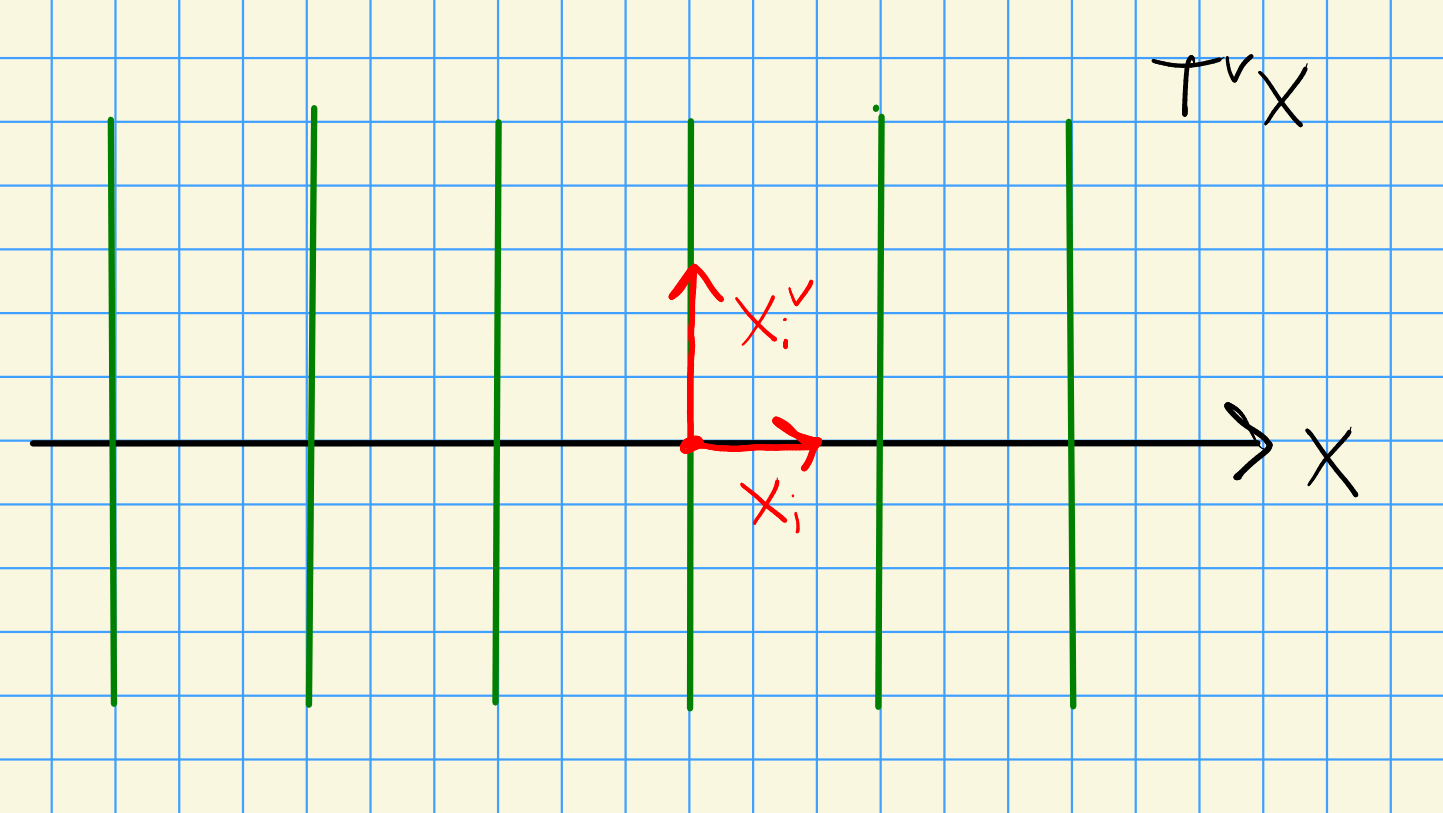So we can write \begin{align*} \lambda = \sum y_i \,dx_i \in \Omega^1({\mathbf{T}} {}^{ \vee }X) ,\end{align*} regarding the $$y_i$$ as functions on $${\mathbf{T}} {}^{ \vee }X$$ and $$\,dx_i$$ as 1-forms on $${\mathbf{T}} {}^{ \vee }X$$.

Find out what $$\beta = \sum a_i \,dx_i$$ is equal to as a section of $${\mathbf{T}} {}^{ \vee }X$$.

To get a contact manifold of dimension $$2n+1$$, consider the 1-jet space $$J^1(X) \mathrel{\vcenter{:}}= T {}^{ \vee }X \times {\mathbb{R}}$$. Write the coordinates as $$(x,y)\in {\mathbf{T}} {}^{ \vee }X$$ and $$z\in {\mathbb{R}}$$ and define $$\alpha = \,dz- \lambda$$, the claim is that this is contact.

For dimension $$2n-1$$, choose a cometric on $$X$$ and take $${\mathbb{S}}{\mathbf{T}} {}^{ \vee }X$$ the unit cotangent bundle of unit-length covectors. Then $$\alpha \mathrel{\vcenter{:}}=-{ \left.{{\lambda}} \right|_{{{\mathbb{S}}{\mathbf{T}} {}^{ \vee }X}} }$$ is contact.

Check that $${\mathbb{R}}^3 = J^1({\mathbb{R}})$$ and $${\mathbb{S}}{\mathbf{T}} {}^{ \vee }({\mathbb{R}}^2) = {\mathbb{R}}^2 \times S^1$$.

A neat theorem: the contact geometry of $${\mathbb{S}}{\mathbf{T}} {}^{ \vee }{\mathbb{R}}^3$$ is a perfect knot invariant. This involves assigning to knots unique Legendrian submanifolds.

## 2.2 Perturbing Foliation

Define \begin{align*} \alpha_t = \,dz- ty\,dx\qquad t\in {\mathbb{R}} \end{align*} to get a 1-parameter family of 1-forms. Check that $$\alpha_t \wedge d \alpha_t = t(\,dz\wedge \,dx\wedge \,dy)$$. Consider $$t\in (-{\varepsilon}, {\varepsilon})$$:

• $$t>0 \implies \alpha = \,dz- y\,dx$$ yields a positive contact structure,
• $$t>0 \implies \alpha = \,dz$$ is a foliation,
• $$t<0 \implies \alpha = \,dz+ y\,dx$$ is a negative contact structure.

What is a (codimension $$r$$) foliation on an $$n{\hbox{-}}$$manifold? A local diffeomorphism $$U\cong {\mathbb{R}}^n \times {\mathbb{R}}^{n-r}$$ with leaves $${\operatorname{pt}}\times{\mathbb{R}}^{n-r}$$. For example, $${\mathbb{R}}^3\cong {\mathbb{R}}\times{\mathbb{R}}^2$$ with coordinates $$t$$ and $$(x, y)$$. We’re leaving out a lot about how many derivatives one needs here!

For a fiber bundle or vector bundle to admit an interesting foliation, one needs a flat connection.

Any $$\xi \mathrel{\vcenter{:}}=\ker \alpha$$ is integrable iff for all vector fields $$X, Y \subseteq \xi$$, their Lie bracket $$[X, Y] \subseteq \xi$$.

For $$\alpha$$ nonvanishing on $$Y^3$$, $$\ker \alpha$$ is tangent to a foliation by surfaces iff $$\alpha \wedge d\alpha = 0$$.

Consider $$\alpha = \,dz- y\,dx$$, so $$\ker \alpha = \mathop{\mathrm{span}}_{\mathbb{R}}\left\{{{\partial}y, y{\partial}z + {\partial}x}\right\}$$ which bracket to $${\partial}z \not \in \ker \alpha$$. This yields a non-integrable contact structure.

On the other hand, for $$\alpha = \,dz$$, $$\ker \alpha = \mathop{\mathrm{span}}_{\mathbb{R}}\left\{{{\partial}x, {\partial}y}\right\}$$ which bracket to zero. So this yields a foliation.

A theorem of Eliashberg and Thurston: taut foliations can be perturbed to a (tight) positive contact structure.

# 3 Tuesday, January 18

Refs:

• Geiges, Intro to Contact
• Ozbogi-Stipsicz
• Etnyre lecture notes
• Massot
• Sivck

For $$S^3 \subseteq {\mathbb{C}}^2$$, define a form on $${\mathbb{R}}^4$$ as \begin{align*} \alpha \mathrel{\vcenter{:}}=-y_1 dx_1 + x_1 dy_1 - y_2 dx_2 + x_2 dy_2 .\end{align*} Then then standard contact form on $$S^3$$ is \begin{align*} \xi_{\text{std}} \mathrel{\vcenter{:}}=\ker { \left.{{\alpha}} \right|_{{S^3}} } .\end{align*}

Show that $$\alpha$$ defines a contact form.

Write $$f = x_1^2 + y_1^2 + x_2^2 + y_2^2$$, then \begin{align*} { \left.{{ \alpha}} \right|_{{S^3}} } \wedge { \left.{{ d\alpha}} \right|_{{S^3}} } > 0 \iff df\wedge d \alpha \wedge d \alpha > 0 .\end{align*}

Check that

• $$d\alpha = 2(dx_1 \wedge dy_1) + 2(dx_2 + dy_2)$$
• $$df = 2(x_1 dx_1 + y_1 dy_1) + 2(x_2 dx_2 + y_2 dy_2)$$.

Note that at $$p= {\left[ {1,0,0,0} \right]} \subseteq S^3$$, $${\mathbf{T}}_pS^3 = \mathop{\mathrm{span}}\left\{{{\partial}y_1, {\partial}x_2, {\partial}y_2}\right\}$$. and $$\alpha_p = -0 dx_1 + 1dy_1 -0 dx_2 + 0dy_2 = dy_1$$ and $$\xi_p = \ker dy_1 = \mathop{\mathrm{span}}\left\{{{\partial}x_1, {\partial}y_2}\right\}$$.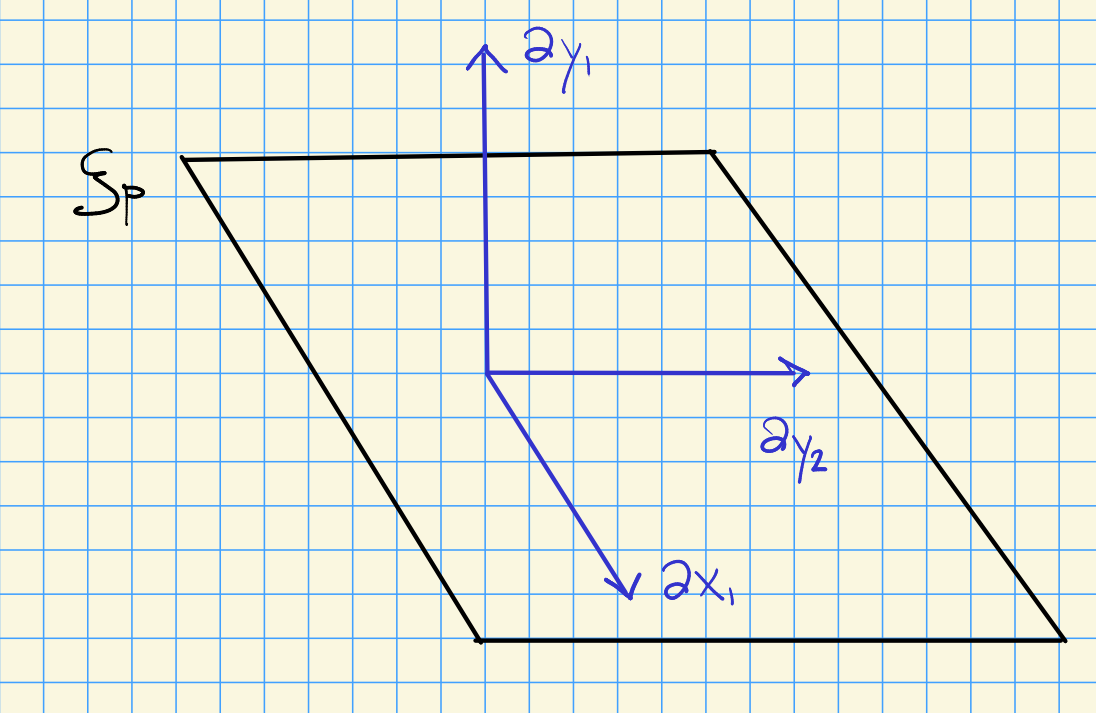Then $$\xi_p \leq {\mathbf{T}}_p {\mathbb{C}}^2 = \mathop{\mathrm{span}}\left\{{{\partial}x_1, {\partial}y_1, {\partial}x_2, {\partial}y_2}\right\} \cong {\mathbb{C}}^4$$ is a distinguished complex line.

An almost complex structure on $$X$$ is a bundle automorphism $$J: {\mathbf{T}}X{\circlearrowleft}$$ with $$J^2 = -\operatorname{id}$$.

For $$X = {\mathbb{C}}^2$$, take \begin{align*} {\partial}x_1 &\mapsto {\partial}y_1 \\ {\partial}y_1 &\mapsto - {\partial}x_1 \\ {\partial}x_2 &\mapsto {\partial}y_2 \\ {\partial}y_2 &\mapsto -{\partial}x_2 .\end{align*}

Show that $$f: {\mathbb{C}}\to {\mathbb{C}}$$ is holomorphic if $$df \circ J = J \circ df$$, which corresponds to the Cauchy-Riemann equations.

Given $$J:W\to W$$, an $${\mathbb{R}}{\hbox{-}}$$subspace $$V \leq W$$ is a $${\mathbb{C}}{\hbox{-}}$$subspace iff $$J(V) = V$$.

The field of $$J{\hbox{-}}$$complex tangents is the hyperplane field \begin{align*} \xi_p \mathrel{\vcenter{:}}={\mathbf{T}}S^3 \cap J({\mathbf{T}}S^3) .\end{align*}

Consider $${\mathbf{T}}_p S^3$$ for $$p={\left[ {1,0,0,0} \right]}$$, then \begin{align*} J(\mathop{\mathrm{span}}\left\{{{\partial}y_1, {\partial}x_1, {\partial}y_2}\right\}) = \mathop{\mathrm{span}}\left\{{-{\partial}x_1, {\partial}y_2, -{\partial}x_2}\right\} ,\end{align*} so $$\xi_p = \mathop{\mathrm{span}}{ {\partial}x_1, {\partial}y_2}$$ is the intersection and coincides $$\xi_{\text{std}}$$.

Where does $$\alpha$$ come from?

Let $$\rho = \sum x_i {\partial}x_i + \sum y_i {\partial}y_i$$ be the radial vector field, so $$\rho = {1\over 2}\operatorname{grad}{\left[ {\sum x_i^2 + \sum y_i^2} \right]}$$. Setting $$\omega \mathrel{\vcenter{:}}=\bigwedge dx_i \wedge \bigwedge dy_i$$, then $$\alpha = \iota_p\omega \mathrel{\vcenter{:}}=\omega(p, {-})$$ is the interior product of $$\omega$$. Then \begin{align*} \alpha = dx_1 \wedge dy_1 (x_1 {\partial}x_1 + y_1 {\partial}y_1 + \cdots ) + \cdots = x_1 dy_1 -y_1 dx_1 + \cdots .\end{align*} So the contact form comes from pairing the symplectic form against a radial vector field.

Recall $$f \mathrel{\vcenter{:}}=\sum x_i^2 + \sum y_i^2$$ satisfies $$df = 2\sum x_i dx_i + 2\sum y_i dy_i$$. Note that $$J$$ acts on 1-forms by $$J^*(dx)({-}) = dx(J({-}))$$. For $$J = i$$,

• $$\delta x: dx(J {\partial}x) = dx({\partial}y) = 0$$,
• $${\partial}y: dx(J {\partial}y) = dx(-{\partial}x) = -1$$.

So $$J^*(dx) = -dy$$, and \begin{align*} J^*(df) = 2x_1 (-dy_1) + 2y_1 (dx_1) + 2x_2 (-dy_2) + 2y_2 (dx_2) = -2 \alpha .\end{align*}

Thus $$J^*(df)$$ is a rotation of $$df$$ by $$\pi/2$$.

The field of complex tangencies along $$Y = f^{-1}(0)$$ is the kernel of $${ \left.{{ df(J({-})) }} \right|_{{Y}} }$$.

Methods of getting contact structures: for a vector field $$X$$, being contact comes from $${\mathcal{L}}_X \omega = \omega$$. For functions $$f:{\mathbb{C}}^2 \to {\mathbb{R}}$$, being contact comes from $$\alpha = d^{\mathbb{C}}f$$ being contact. See strictly plurisubharmonic functions and Levi pseudoconvex subspaces.

The standard contact structure is orthogonal to the Hopf fibration: define a map \begin{align*} {\mathbb{C}}^2\setminus\left\{{0}\right\}&\to {\mathbb{CP}}^1 \cong S^2 \\ {\left[ {z, w} \right]} &\mapsto {\left[ {z: w} \right]} ,\end{align*} which restricts to a map $$S^3\to S^2$$ defining the Hopf fibration. If $$L$$ is a complex line through 0, then $$L \cap S^3$$ is a Hopf fiber that is homeomorphic to $$S^1$$.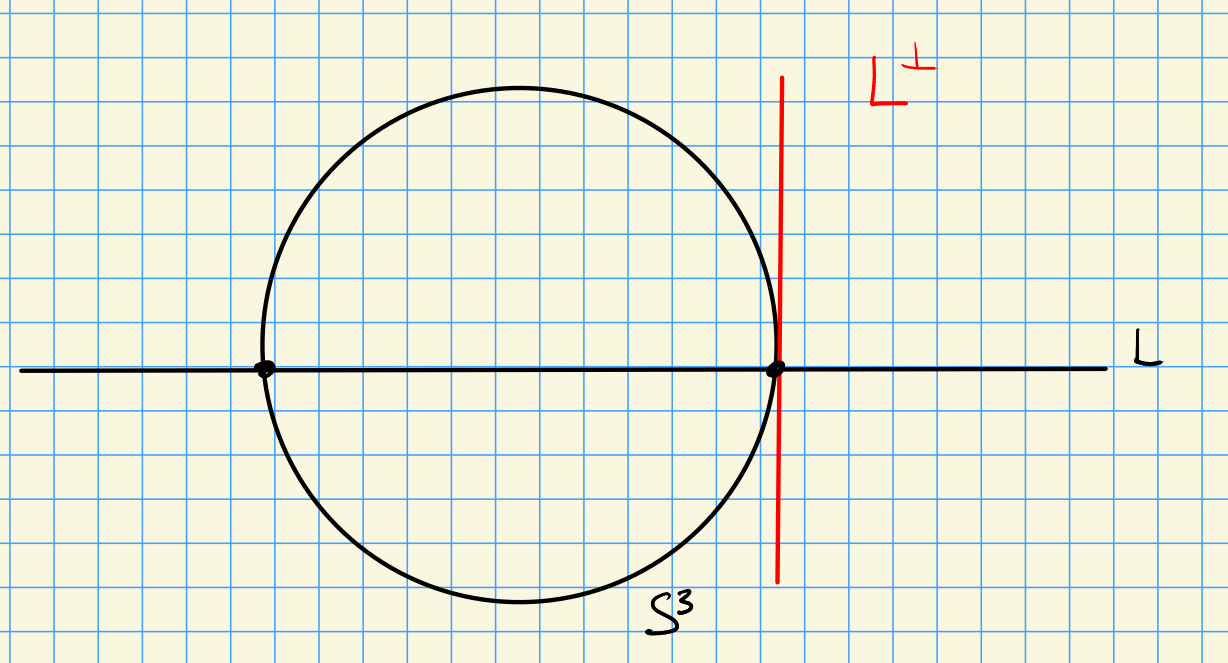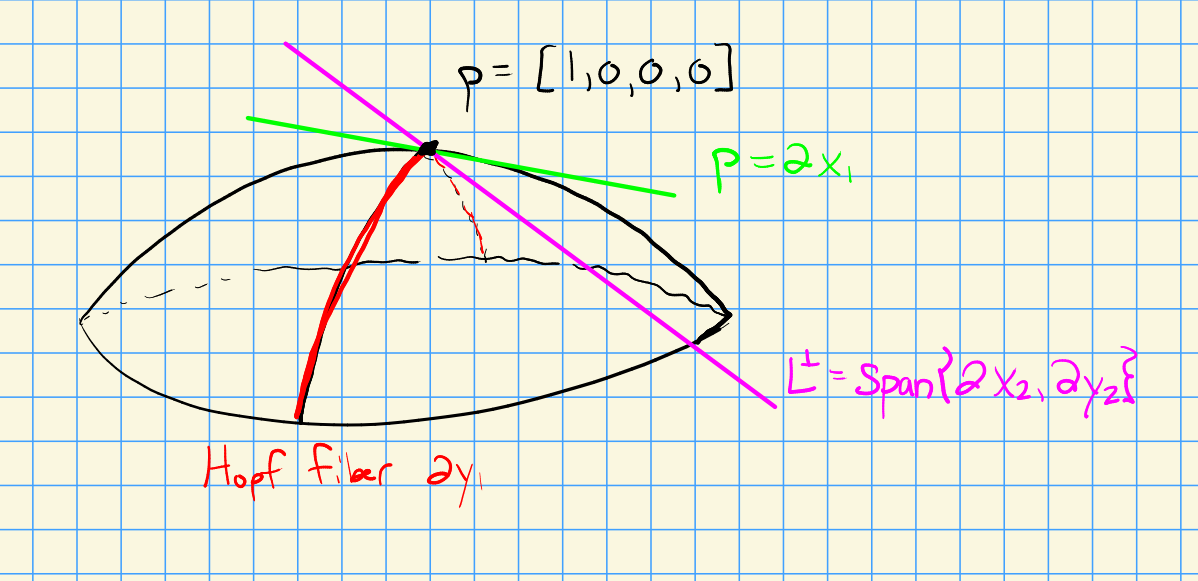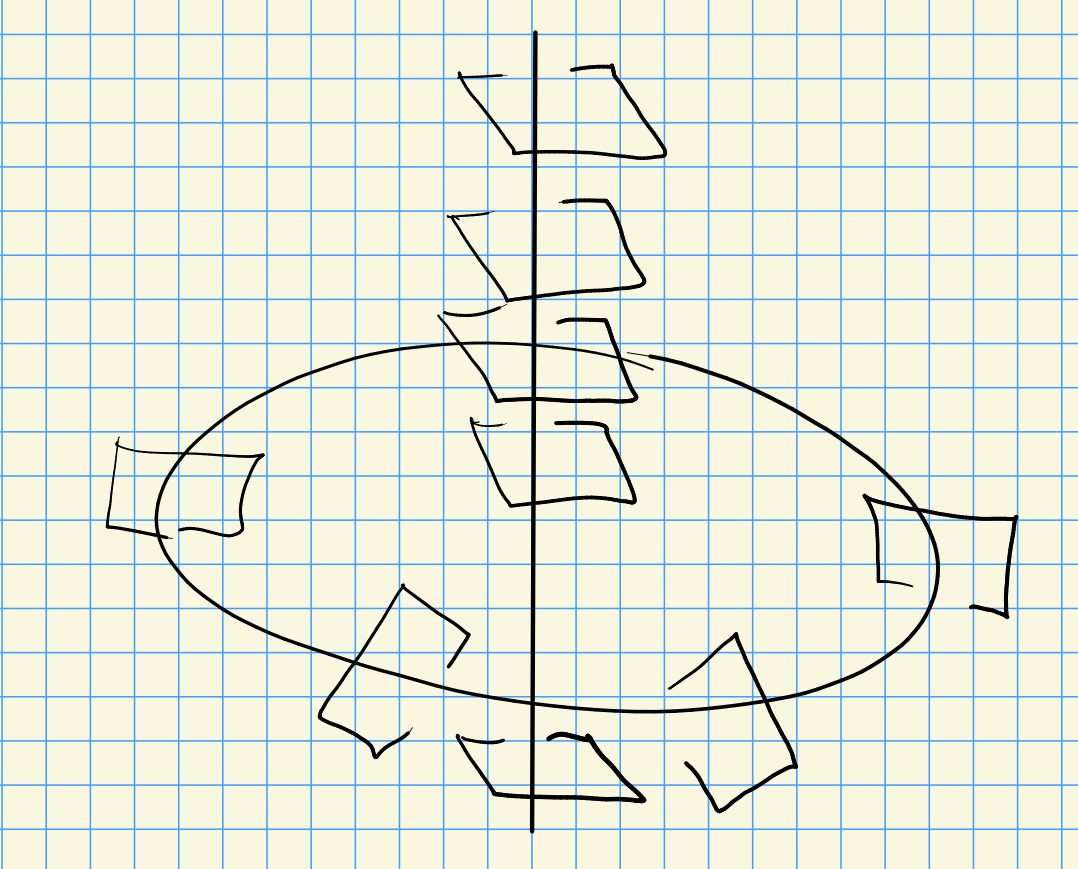Take \begin{align*} {\mathbb{C}}^2 &\to {\mathbb{R}}^2 \\ (z_1, z_2)&\mapsto ({\left\lvert {z_1} \right\rvert}, {\left\lvert {z_2} \right\rvert}) .\end{align*} Consider the image of $$S^2 = \left\{{{\left\lvert {z_1} \right\rvert}^2 + {\left\lvert {z_2} \right\rvert}^2 = 1}\right\}$$: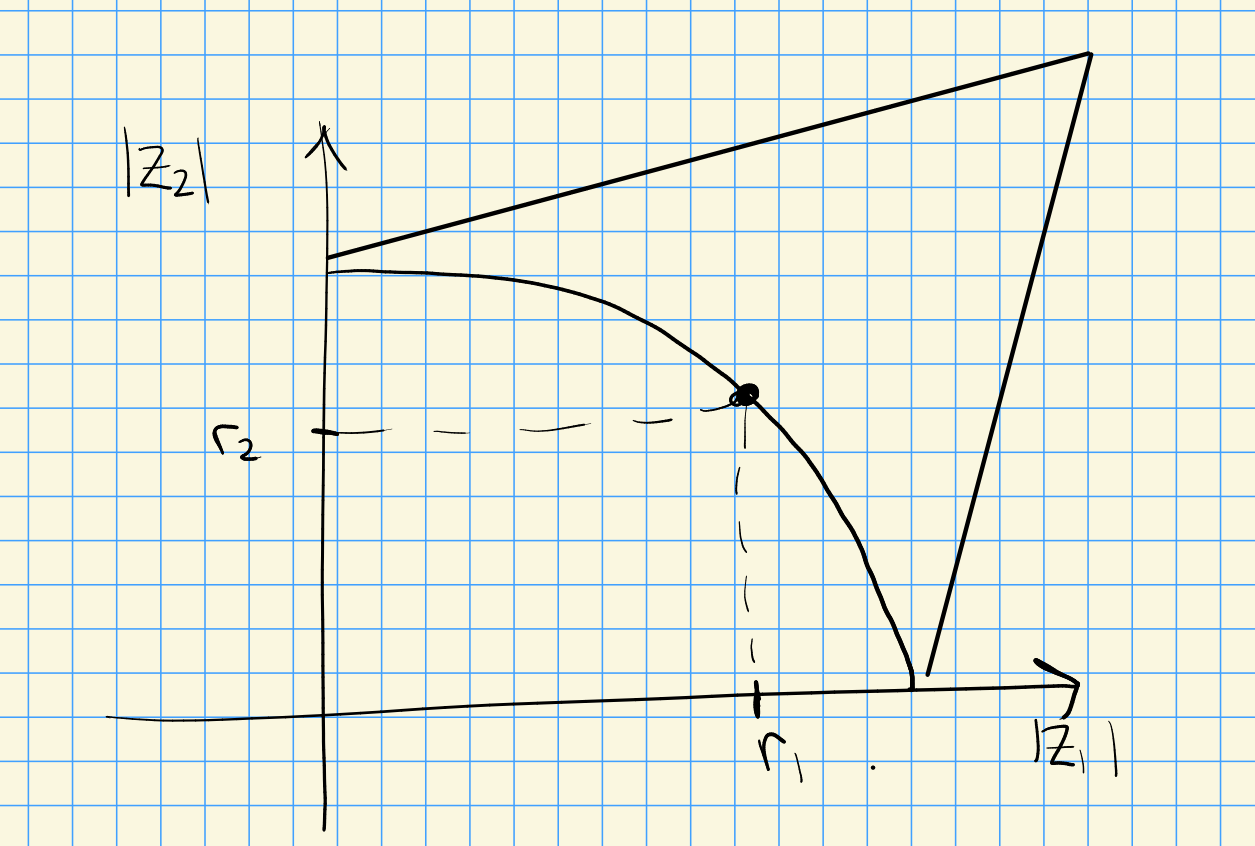The preimage is $$S^1\times S^1$$. This can be realized as a tetrahedron with sides identified: